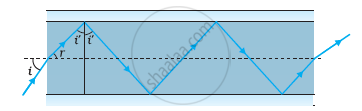# Shows a Cross-section of a ‘Light Pipe’ Made of a Glass Fibre of Refractive Index 1.68. the Outer Covering of the Pipe is - Physics

Numerical

(a) Figure shows a cross-section of a ‘light pipe’ made of a glass fibre of refractive index 1.68. The outer covering of the pipe is made of a material of refractive index 1.44. What is the range of the angles of the incident rays with the axis of the pipe for which total reflections inside the pipe take place, as shown in the figure?

(b) What is the answer if there is no outer covering of the pipe?#### Solution

(a) Refractive index of the glass fibre, μ1 = 1.68

Refractive index of the outer covering of the pipe, μ2 = 1.44

Angle of incidence = i

Angle of refraction = r

Angle of incidence at the interface = i'

The refractive index (μ) of the inner core − outer core interface is given as:

μ = μ_2/μ_1 = 1/(sin "i'")

sin "i'" = (μ_1)/(μ_2)

= 1.44/1.68

= 0.8571

∴ i' = 59°

For the critical angle, total internal reflection (TIR) takes place only when i > i', i.e., i > 59°

Maximum angle of reflection, rmax = 90° − i' = 90° − 59° = 31°

Let, imax be the maximum angle of incidence.

The refractive index at the air-glass interface, μ1 = 1.68

We have the relation for the maximum angles of incidence and reflection as:

μ_1 = (sin "i"_"max")/sin "r"_"max"

sin imax = μ1 sin rmax

= 1.68 sin 31°

= 1.68 × 0.5150

= 0.8652

∴ imax = sin−1 0.8652 ≈ 60°

Thus, all the rays incident at angles lying in the range 0 < i < 60° will suffer total internal reflection

(b) If the outer covering of the pipe is not present, then:

Refractive index of the outer pipe, μ1 = Refractive index of air = 1

For the angle of incidence i = 90°, we can write Snell’s law at the air − pipe interface as:

sin "i"/sin "r" = μ_2 = 1.68

sin "r" = (sin 90°)/1.68 = 1/1.68

"r" = sin^(-1) (0.5952)

= 36.5°

∴ i' = 90° − 36.5° = 53.5°

Since i' > r, all incident rays will suffer total internal reflection.

Concept: Refraction
Is there an error in this question or solution?

#### APPEARS IN

NCERT Physics Part 1 and 2 Class 12
Chapter 9 Ray Optics and Optical Instruments
Exercise | Q 9.17 | Page 345
NCERT Class 12 Physics Textbook
Chapter 9 Ray Optics and Optical Instruments
Exercise | Q 17 | Page 347

Share